# 贝叶斯定理的简单证明

/ 1评 / 8

## 证明

### 过程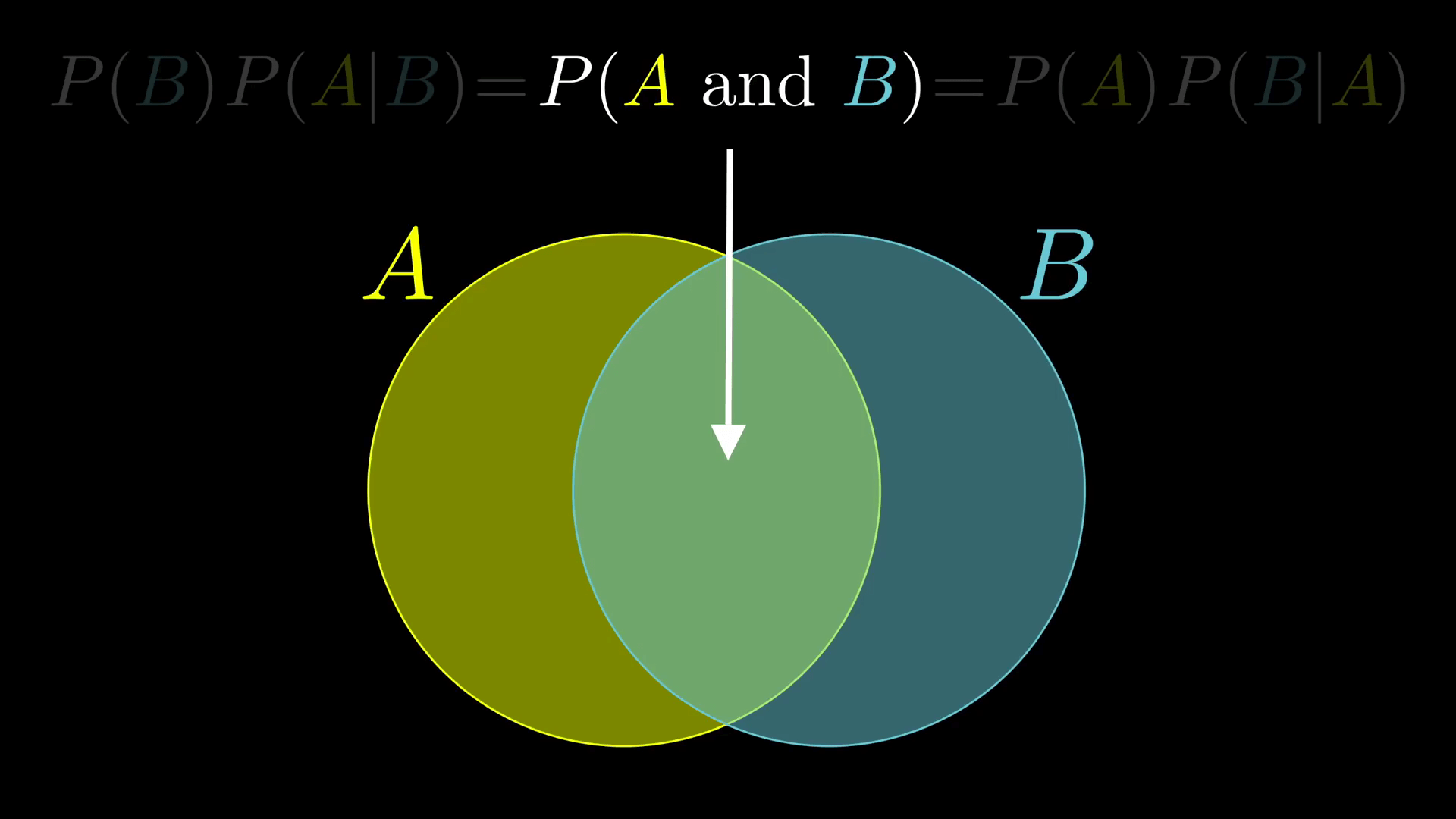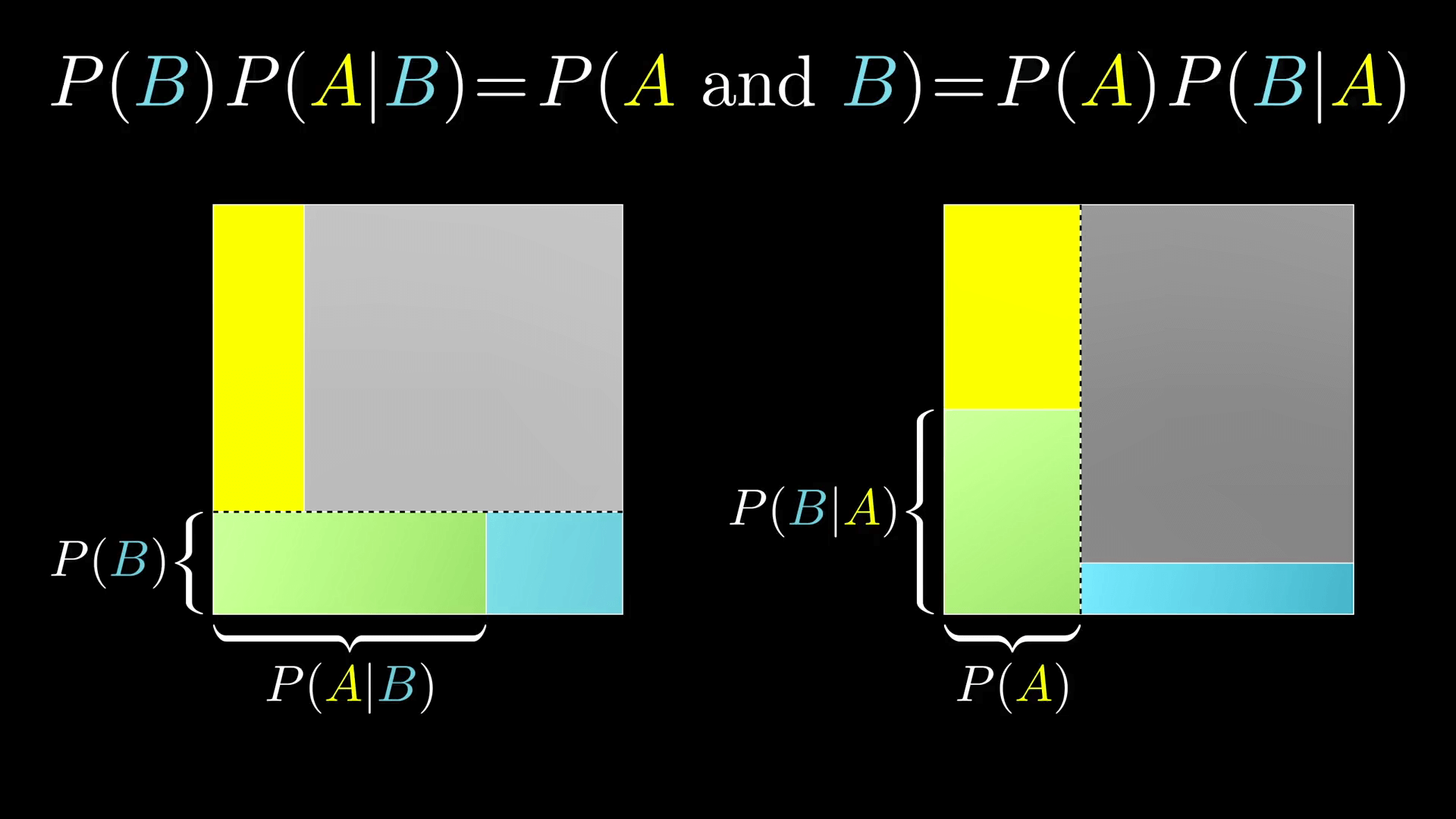P(A \ and \ B) = P(B)P(A|B) = P(A)P(B|A)

P(A|B) = \frac{P(A)P(B|A)}{P(B)}

P(B|A) = \frac{P(B)P(A|B)}{P(A)}

### 补充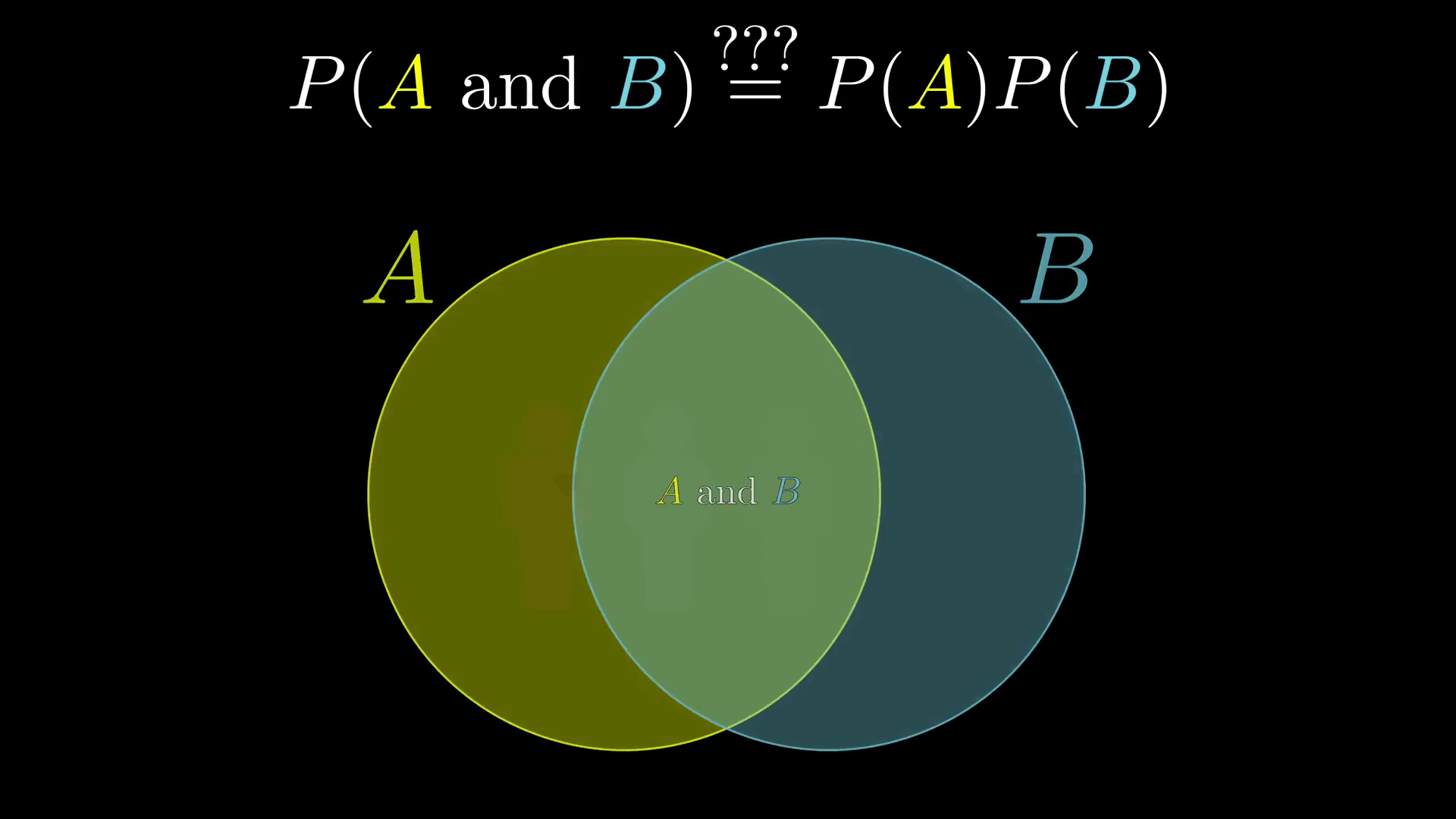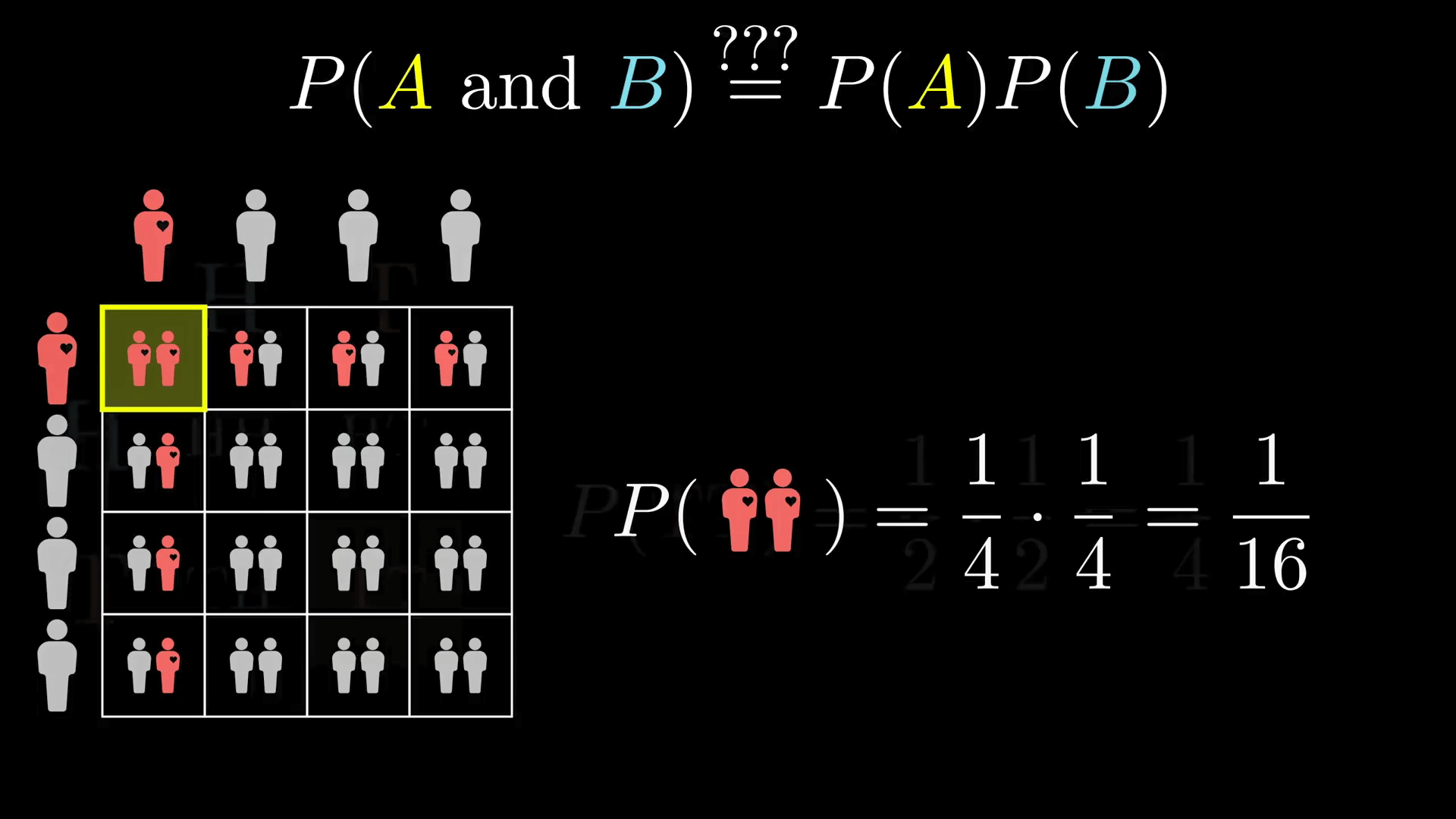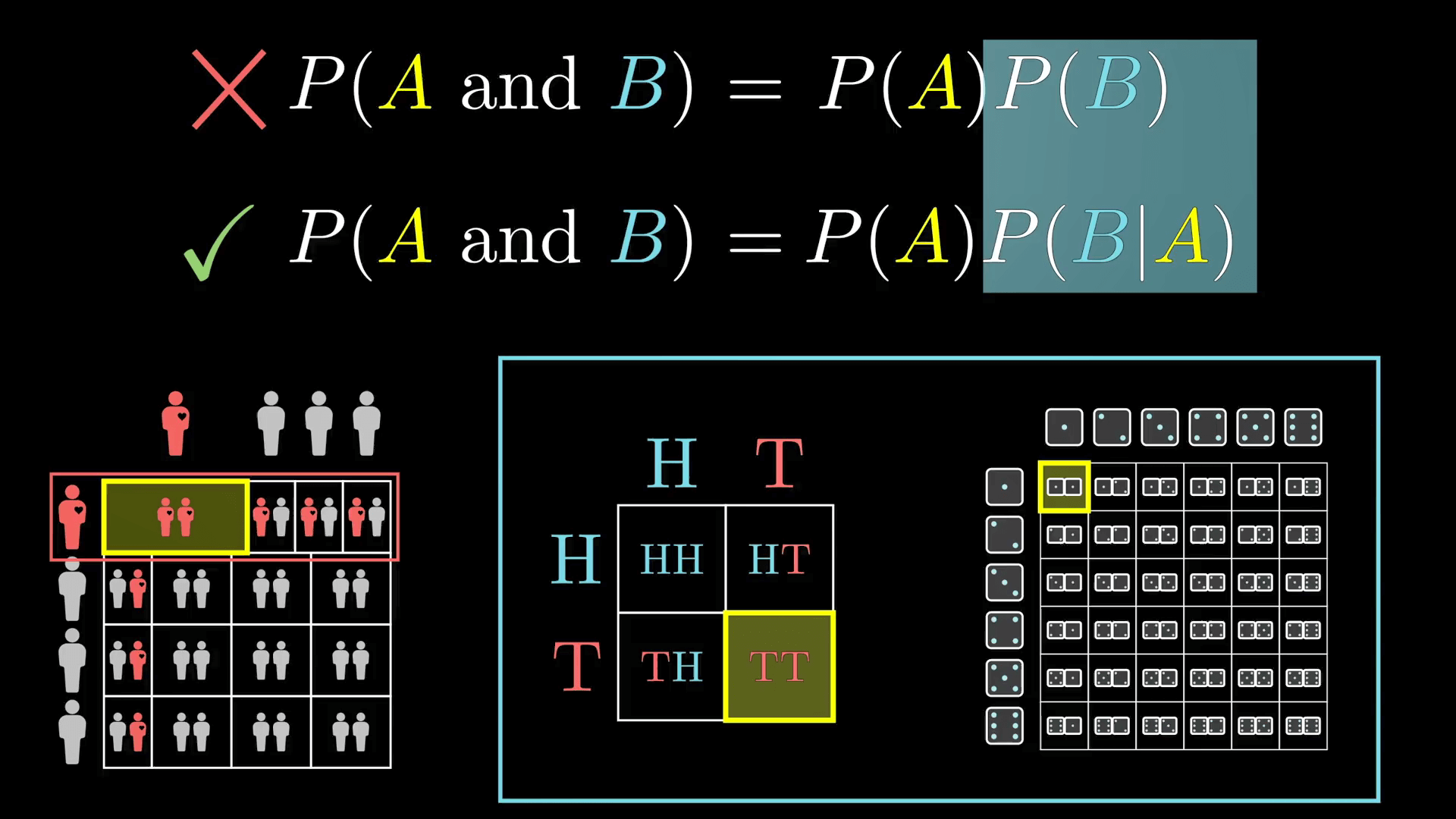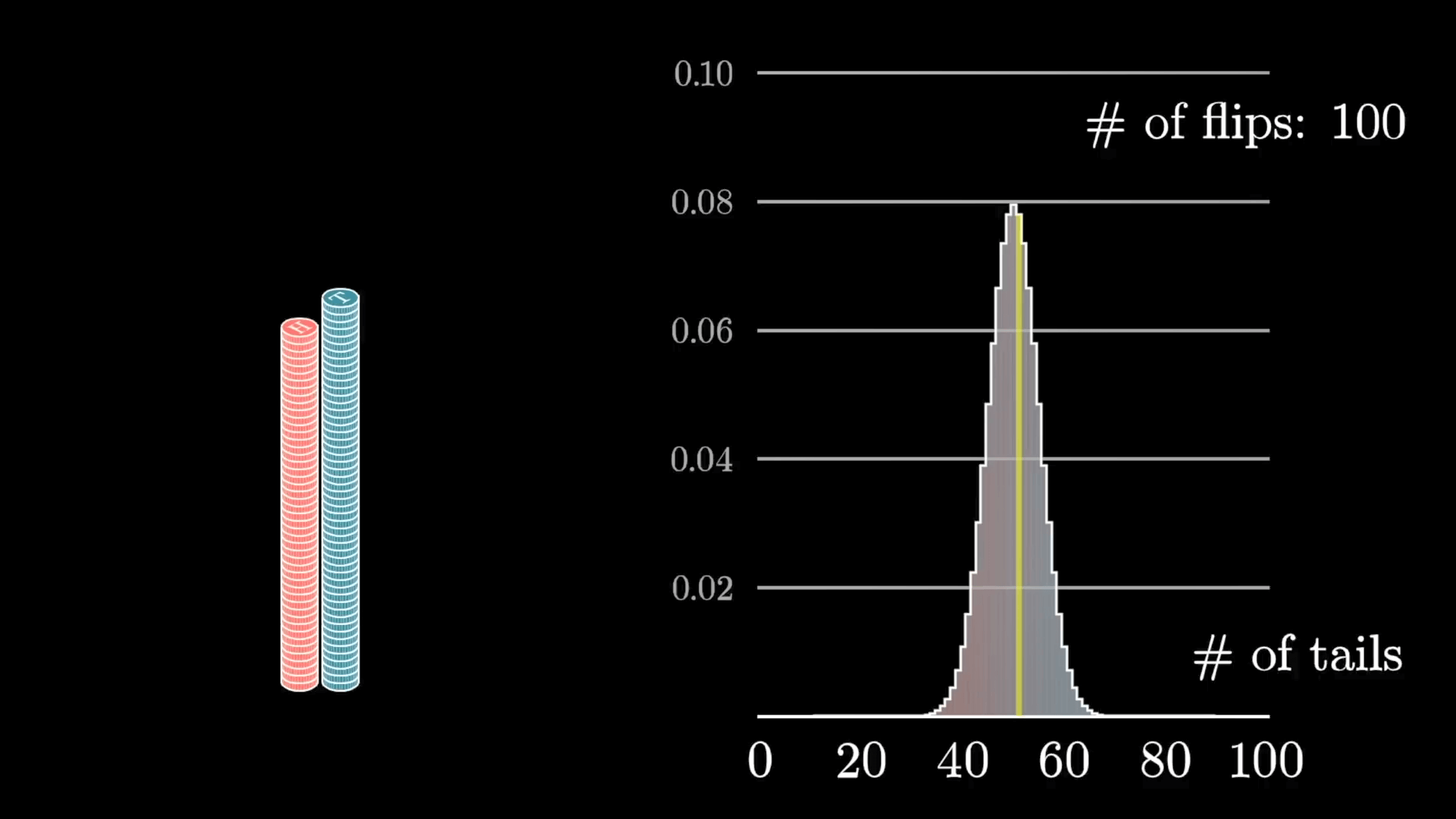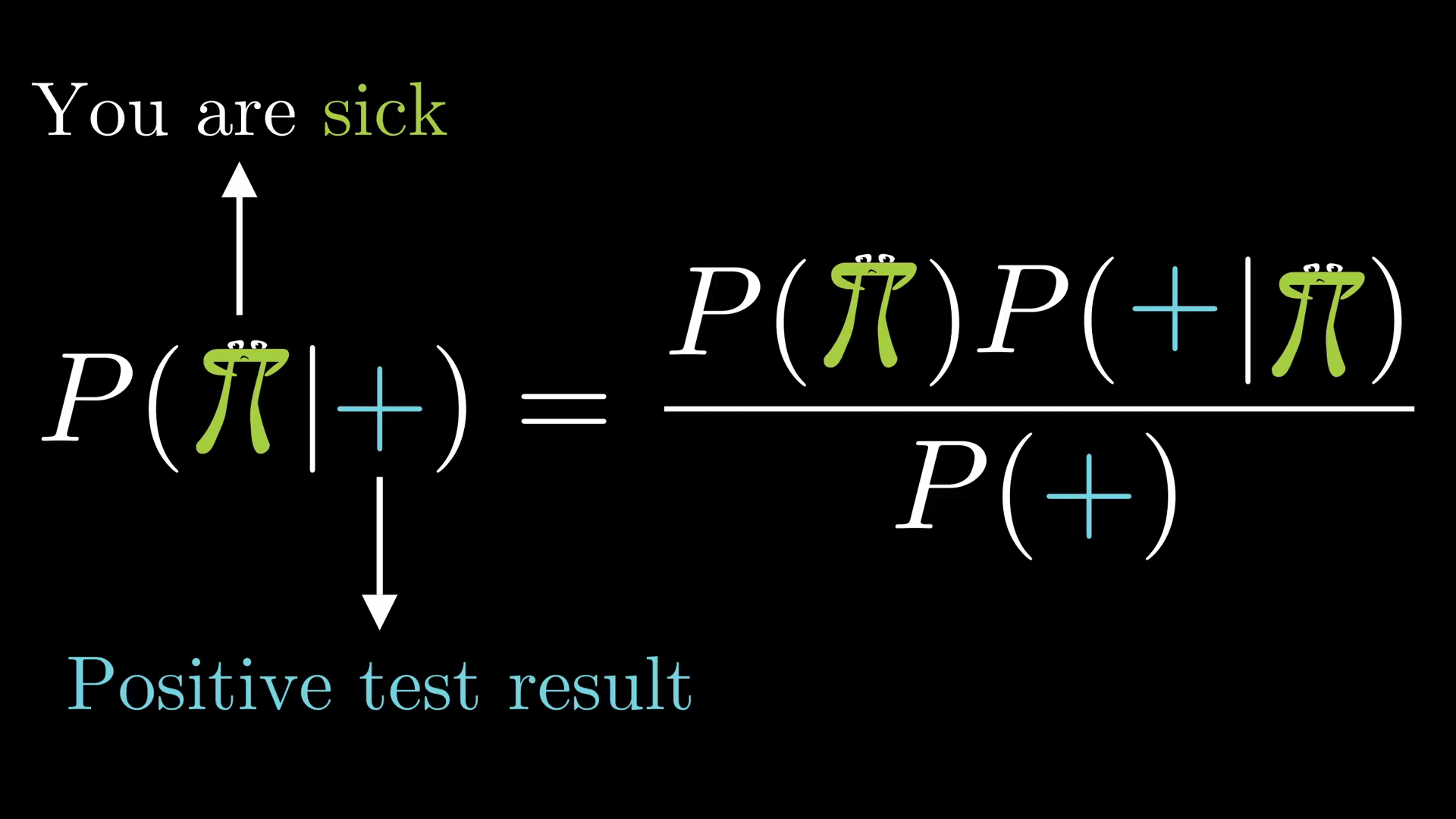## 例题

### 描述

Steve（男性）在准确率为 90% 的男性膀胱癌检测中，其检测结果呈阳性，请问这能说明 Steve 有 90% 的概率患膀胱癌么？

### 求解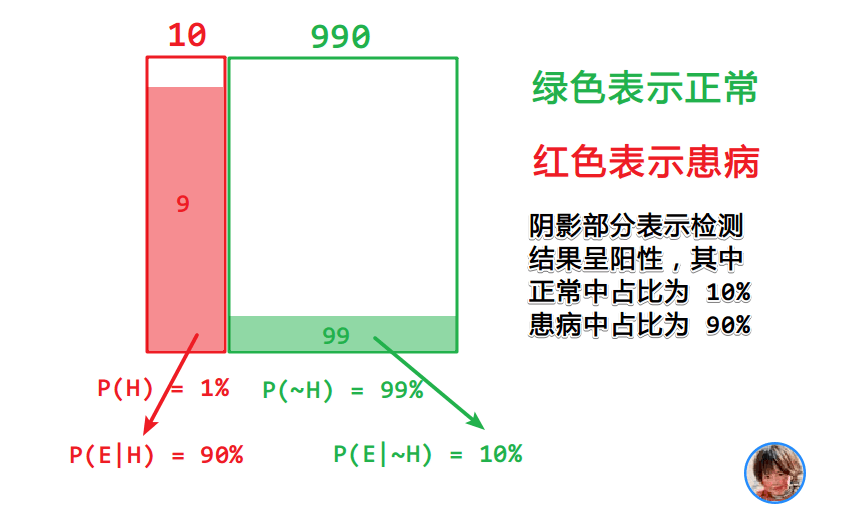P(H|E) = \frac{P(H)P(E|H)}{P(E)} = \frac{0.01 \times 0.9}{0.01 \times 0.9 + 0.99 \times 0.1} ≈ 0.083

1.CongTsang说道：

这集我看过 😛 经典输入法水印，i了i了

• 默认
• 护眼
• 夜晚
• Serif
• Sans